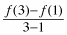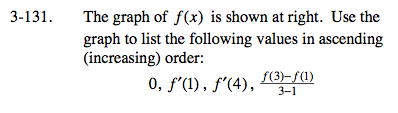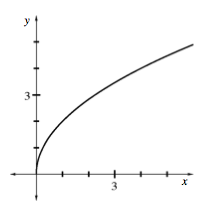### Home > CALC > Chapter Ch3 > Lesson 3.3.4 > Problem3-131

3-131.
1. The graph of f(x) is shown at right. Use the graph to list the following values in ascending (increasing) order:

0, f ′(1), f ′(4),2. 3-131 HW eTool (Desmos). Homework Help ✎f '(1) means the slope at x = 1. Is the slope at x = 1 positive, negative or zero? How does this compare with the slope at x = 4?

f '(4) means the slope at x = 4. Is the slope at x = 4 positive, negative or zero? How does it compare with the slope at x = 1?means the average rate of change between x = 3 and x = 1.

Graphically, this can be seen as the slope of the secant line. Is that slope positive, negative or zero? How does it compare with the slopes at x = 1 and x = 4, and with the value of 0?

Looking at the graph from left to right, it is increasing always. The slopes start steep and, as x→∞, get smoother.

Use the eTool below to help solve the problem.
Click the link at right for the full version of the eTool: Calc 3-131 HW eTool